Help protect the Great Barrier Reef with TensorFlow on Kaggle

Boosted trees using Estimators

This tutorial is an end-to-end walkthrough of training a Gradient Boosting model using decision trees with the tf.estimator API. Boosted Trees models are among the most popular and effective machine learning approaches for both regression and classification. It is an ensemble technique that combines the predictions from several (think 10s, 100s or even 1000s) tree models.

Boosted Trees models are popular with many machine learning practitioners as they can achieve impressive performance with minimal hyperparameter tuning.

You will be using the titanic dataset, where the (rather morbid) goal is to predict passenger survival, given characteristics such as gender, age, class, etc.

import numpy as np
import pandas as pd
from IPython.display import clear_output
from matplotlib import pyplot as plt

y_train = dftrain.pop('survived')
y_eval = dfeval.pop('survived')
import tensorflow as tf
tf.random.set_seed(123)

The dataset consists of a training set and an evaluation set:

• dftrain and y_train are the training set—the data the model uses to learn.
• The model is tested against the eval set, dfeval, and y_eval.

For training you will use the following features:

Feature Name Description
sex Gender of passenger
age Age of passenger
n_siblings_spouses siblings and partners aboard
parch of parents and children aboard
fare Fare passenger paid.
class Passenger's class on ship
deck Which deck passenger was on
embark_town Which town passenger embarked from
alone If passenger was alone

Explore the data

Let's first preview some of the data and create summary statistics on the training set.

dftrain.describe()

There are 627 and 264 examples in the training and evaluation sets, respectively.

dftrain.shape, dfeval.shape
(627, 264)

The majority of passengers are in their 20's and 30's.

dftrain.age.hist(bins=20)
plt.show()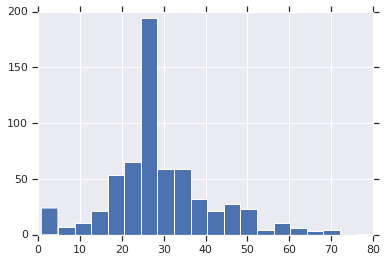There are approximately twice as male passengers as female passengers aboard.

dftrain.sex.value_counts().plot(kind='barh')
plt.show()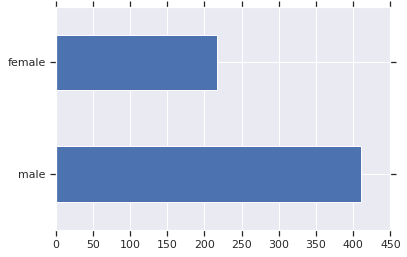The majority of passengers were in the "third" class.

dftrain['class'].value_counts().plot(kind='barh')
plt.show()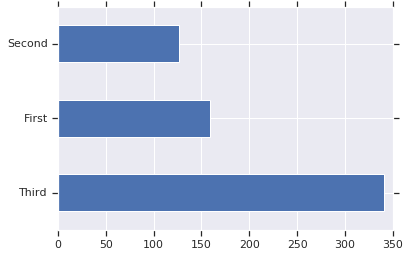Most passengers embarked from Southampton.

dftrain['embark_town'].value_counts().plot(kind='barh')
plt.show()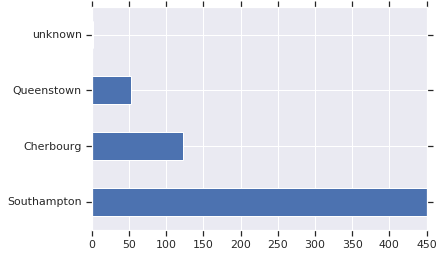Females have a much higher chance of surviving vs. males. This will clearly be a predictive feature for the model.

pd.concat([dftrain, y_train], axis=1).groupby('sex').survived.mean().plot(kind='barh').set_xlabel('% survive')
plt.show()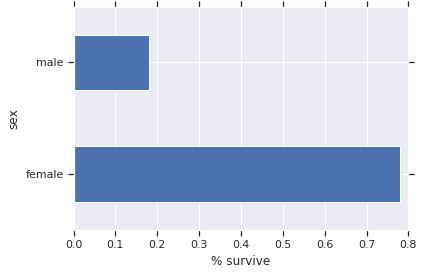Create feature columns and input functions

The Gradient Boosting estimator can utilize both numeric and categorical features. Feature columns work with all TensorFlow estimators and their purpose is to define the features used for modeling. Additionally they provide some feature engineering capabilities like one-hot-encoding, normalization, and bucketization. In this tutorial, the fields in CATEGORICAL_COLUMNS are transformed from categorical columns to one-hot-encoded columns (indicator column):

CATEGORICAL_COLUMNS = ['sex', 'n_siblings_spouses', 'parch', 'class', 'deck',
'embark_town', 'alone']
NUMERIC_COLUMNS = ['age', 'fare']

def one_hot_cat_column(feature_name, vocab):
return tf.feature_column.indicator_column(
tf.feature_column.categorical_column_with_vocabulary_list(feature_name,
vocab))
feature_columns = []
for feature_name in CATEGORICAL_COLUMNS:
# Need to one-hot encode categorical features.
vocabulary = dftrain[feature_name].unique()
feature_columns.append(one_hot_cat_column(feature_name, vocabulary))

for feature_name in NUMERIC_COLUMNS:
feature_columns.append(tf.feature_column.numeric_column(feature_name,
dtype=tf.float32))

You can view the transformation that a feature column produces. For example, here is the output when using the indicator_column on a single example:

class_fc = tf.feature_column.indicator_column(tf.feature_column.categorical_column_with_vocabulary_list('class', ('First', 'Second', 'Third')))
print('Feature value: "{}"'.format(example['class'].iloc))
print('One-hot encoded: ', tf.keras.layers.DenseFeatures([class_fc])(example).numpy())
Feature value: "Third"
One-hot encoded:  [[0. 0. 1.]]

Additionally, you can view all of the feature column transformations together:

tf.keras.layers.DenseFeatures(feature_columns)(example).numpy()
array([[22.  ,  1.  ,  0.  ,  1.  ,  0.  ,  0.  ,  1.  ,  0.  ,  0.  ,

0.  ,  0.  ,  0.  ,  0.  ,  0.  ,  1.  ,  0.  ,  0.  ,  0.  ,
7.25,  1.  ,  0.  ,  0.  ,  0.  ,  0.  ,  0.  ,  0.  ,  1.  ,
0.  ,  0.  ,  0.  ,  0.  ,  0.  ,  1.  ,  0.  ]], dtype=float32)

Next you need to create the input functions. These will specify how data will be read into our model for both training and inference. You will use the from_tensor_slices method in the tf.data API to read in data directly from Pandas. This is suitable for smaller, in-memory datasets. For larger datasets, the tf.data API supports a variety of file formats (including csv) so that you can process datasets that do not fit in memory.

# Use entire batch since this is such a small dataset.
NUM_EXAMPLES = len(y_train)

def make_input_fn(X, y, n_epochs=None, shuffle=True):
def input_fn():
dataset = tf.data.Dataset.from_tensor_slices((dict(X), y))
if shuffle:
dataset = dataset.shuffle(NUM_EXAMPLES)
# For training, cycle thru dataset as many times as need (n_epochs=None).
dataset = dataset.repeat(n_epochs)
# In memory training doesn't use batching.
dataset = dataset.batch(NUM_EXAMPLES)
return dataset
return input_fn

# Training and evaluation input functions.
train_input_fn = make_input_fn(dftrain, y_train)
eval_input_fn = make_input_fn(dfeval, y_eval, shuffle=False, n_epochs=1)

Train and evaluate the model

Below you will do the following steps:

1. Initialize the model, specifying the features and hyperparameters.
2. Feed the training data to the model using the train_input_fn and train the model using the train function.
3. You will assess model performance using the evaluation set—in this example, the dfeval DataFrame. You will verify that the predictions match the labels from the y_eval array.

Before training a Boosted Trees model, let's first train a linear classifier (logistic regression model). It is best practice to start with a simpler model to establish a benchmark.

linear_est = tf.estimator.LinearClassifier(feature_columns)

# Train model.
linear_est.train(train_input_fn, max_steps=100)

# Evaluation.
result = linear_est.evaluate(eval_input_fn)
clear_output()
print(pd.Series(result))
accuracy                  0.765152
accuracy_baseline         0.625000
auc                       0.832844
auc_precision_recall      0.789631
average_loss              0.478908
label/mean                0.375000
loss                      0.478908
precision                 0.703297
prediction/mean           0.350790
recall                    0.646465
global_step             100.000000
dtype: float64

Next let's train a Boosted Trees model. For boosted trees, regression (BoostedTreesRegressor) and classification (BoostedTreesClassifier) are supported. Since the goal is to predict a class - survive or not survive, you will use the BoostedTreesClassifier.

# Since data fits into memory, use entire dataset per layer. It will be faster.
# Above one batch is defined as the entire dataset.
n_batches = 1
est = tf.estimator.BoostedTreesClassifier(feature_columns,
n_batches_per_layer=n_batches)

# The model will stop training once the specified number of trees is built, not
# based on the number of steps.
est.train(train_input_fn, max_steps=100)

# Eval.
result = est.evaluate(eval_input_fn)
clear_output()
print(pd.Series(result))
accuracy                  0.837121
accuracy_baseline         0.625000
auc                       0.871993
auc_precision_recall      0.858760
average_loss              0.406563
label/mean                0.375000
loss                      0.406563
precision                 0.797872
prediction/mean           0.384452
recall                    0.757576
global_step             100.000000
dtype: float64

Now you can use the train model to make predictions on a passenger from the evaluation set. TensorFlow models are optimized to make predictions on a batch, or collection, of examples at once. Earlier, the eval_input_fn is defined using the entire evaluation set.

pred_dicts = list(est.predict(eval_input_fn))
probs = pd.Series([pred['probabilities'] for pred in pred_dicts])

probs.plot(kind='hist', bins=20, title='predicted probabilities')
plt.show()
INFO:tensorflow:Calling model_fn.
INFO:tensorflow:Done calling model_fn.
INFO:tensorflow:Graph was finalized.
INFO:tensorflow:Restoring parameters from /tmp/tmp98s7_n3y/model.ckpt-100
INFO:tensorflow:Running local_init_op.
INFO:tensorflow:Done running local_init_op.
Exception ignored in: <function CapturableResource.__del__ at 0x7ff2fb5bd950>
Traceback (most recent call last):
File "/tmpfs/src/tf_docs_env/lib/python3.7/site-packages/tensorflow/python/training/tracking/tracking.py", line 269, in __del__
with self._destruction_context():
AttributeError: 'TreeEnsemble' object has no attribute '_destruction_context'Finally you can also look at the receiver operating characteristic (ROC) of the results, which will give us a better idea of the tradeoff between the true positive rate and false positive rate.

from sklearn.metrics import roc_curve

fpr, tpr, _ = roc_curve(y_eval, probs)
plt.plot(fpr, tpr)
plt.title('ROC curve')
plt.xlabel('false positive rate')
plt.ylabel('true positive rate')
plt.xlim(0,)
plt.ylim(0,)
plt.show()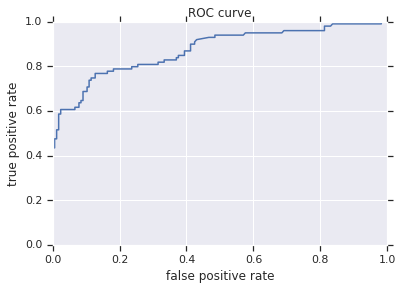[]
[]